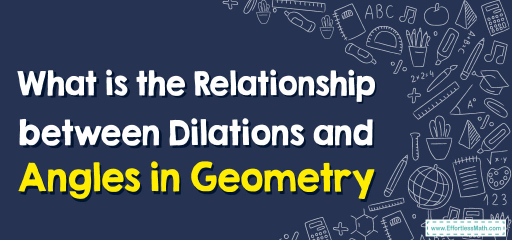# What is the Relationship between Dilations and Angles in Geometry

While dilation changes the size of a figure, it does so proportionally, ensuring the figure's shape remains unchanged. An intriguing consequence of this proportionality is that angles within the figure remain consistent post-dilation. Essentially, regardless of how much we stretch or compress a figure through dilation, its angles remain steadfast and unchanged. This characteristic has pivotal implications in geometry, especially when proving the similarity of shapes.## Step-by-step Guide: Dilations and Angles

1. Understanding Dilation:
Remember, dilation is a transformation that proportionally increases or decreases the size of a figure based on a scale factor. The key here is proportionality.
2. Angle Preservation:
Despite the lengths of the sides undergoing changes during dilation, angles remain unaltered. This is because all sides are stretched or compressed by the same factor, ensuring that the shape and angles are preserved.
3. Implications for Triangles and Polygons:
For triangles and polygons, if one is a dilation of the other, then they are similar. Their corresponding angles are congruent, and their sides are proportional.

### Examples

Example 1:
Triangle $$ABC$$ has angles $$\angle A = 60^\circ$$, $$\angle B = 70^\circ$$, and $$\angle C = 50^\circ$$. If Triangle $$A’B’C’$$ is a dilation of Triangle $$ABC$$, what are the angles of Triangle $$A’B’C’$$?

Solution:
Since dilation preserves angles:
$$\angle A’ = \angle A = 60^\circ$$
$$\angle B’ = \angle B = 70^\circ$$
$$\angle C’ = \angle C = 50^\circ$$
Thus, Triangle $$A’B’C’$$ has angles of $$60^\circ$$, $$70^\circ$$, and $$50^\circ$$.

Example 2:
A quadrilateral $$WXYZ$$ has angles $$\angle W = 90^\circ$$, $$\angle X = 80^\circ$$, $$\angle Y = 95^\circ$$, and $$\angle Z = 95^\circ$$. If Quadrilateral $$W’X’Y’Z’$$ is a dilation of Quadrilateral $$WXYZ$$, what are the angles of Quadrilateral $$W’X’Y’Z’$$?

Solution:
Given the property of angle preservation in dilation:
$$\angle W’ = \angle W = 90^\circ$$
$$\angle X’ = \angle X = 80^\circ$$
$$\angle Y’ = \angle Y = 95^\circ$$
$$\angle Z’ = \angle Z = 95^\circ$$
Thus, Quadrilateral $$W’X’Y’Z’$$ has angles of $$90^\circ$$, $$80^\circ$$, $$95^\circ$$, and $$95^\circ$$.

### Practice Questions:

1. If $$\angle A$$ in Triangle $$ABC$$ is $$40^\circ$$ and Triangle $$DEF$$ is a dilation of Triangle $$ABC$$, what is $$\angle D$$ in Triangle $$DEF$$?
2. A pentagon $$ABCDE$$ has $$\angle A = 108^\circ$$. If Pentagon $$A’B’C’D’E’$$ is a dilation of Pentagon $$ABCDE$$, what is $$\angle A’$$?

1. $$40^\circ$$
2. $$108^\circ$$

### What people say about "What is the Relationship between Dilations and Angles in Geometry - Effortless Math: We Help Students Learn to LOVE Mathematics"?

No one replied yet.

X
30% OFF

Limited time only!

Save Over 30%

SAVE $5 It was$16.99 now it is \$11.99